(二) 计算方法的详细说明

球体的内部星体较多,球体外部较少,它们的分界处就是球面。
球面邻近的星体形成小宇宙的最外层。

这是观测的结果,如图 1 所示。

宇宙的平均质量密度是 10-30克/厘米3 数量级；
宇宙的半径绝对不大于 6 Gpc;
哈勃常数肯定小于100， 等等。

1. 计算方法的流程

1) 在球面的计算中,获得如下8个参数
H    M    a    m      r     L0     L     Hmax
2) 再借助球外的计算,获得
Zmax
3) 再借助球内的计算,获得
Mv

4) 用计算出的 H    M  r   a     Hmax      Zmax      Mv
我决定一组参数   H     M  r   a       m    Mv
我认为小宇宙就是这样.
我用这些参数去计算  Zr 和  Z―m  关系
最后拟合哈勃图,看这些参数正确否.

2. 球面中的公式有 3 个, 如下: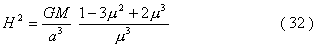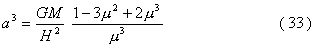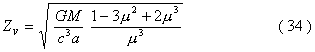把公式(33)代入公式(34),消去 a ,只剩下三个参数

H      M    m

3. 求所有的 H  M m  组合

应用等式(37):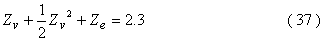得到三元一次不定方程:
f ( M, H, m )  = 2.3

我们任意选定 HM 的数值,例如
M=M1                H=H1
适当选取 m 的数值,使
f  ( M1, H1, m11 ) = 2.3
我们得到一组参数
M1     H1   m11

1) 设 M=M1, H=H2  得到一组参数
M1      H2    m12
2) 设 M=M1  H=H3  得到一组参数
M1       H3    m13
3) 遍历 H ,得

M1       H1    m11
M1       H2     m12
M1       H3     m13
.............
M1       Hn    m1n

4) 设 M=M2,   H=H1,   H=H2,   H=H3, 遍历 H

M2       H1    m21
M2       H2     m22
M2       H3     m23
.............
M2       Hn    m2n

5) 遍历 M 和 H 得到 M,  H, 和 m 的全部组合 Mi  Hij  mij  如表 2 所示(附录十一).

3. 应用公式(33)求出所有的 a , 如表 2 所示(附录十一).

4. 应用公式(17)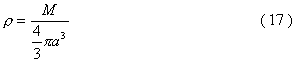求出所有的 r ,如表 2 所示(附录十一).

5. 应用公式(8)和(7)
a0 = ma                           (8)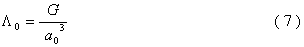求出所有的 L0 ,如表 2 所示(附录十一).

6. 应用公式(10)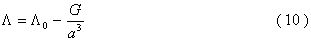7. 应用公式(15)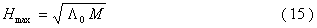8. 借助球外的计算公式求  Zmax
应用公式(29)
Zmax = 1.5 + Ze                  (29)
求出所有的 Zmax ,如表 2 所示(附录十一).
计算步骤如下:
1) 球外的速度红移是公式(24):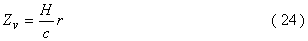相对论修正项为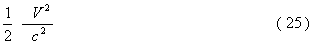总速度红移为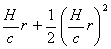球外的斥力红移是公式(第42页):